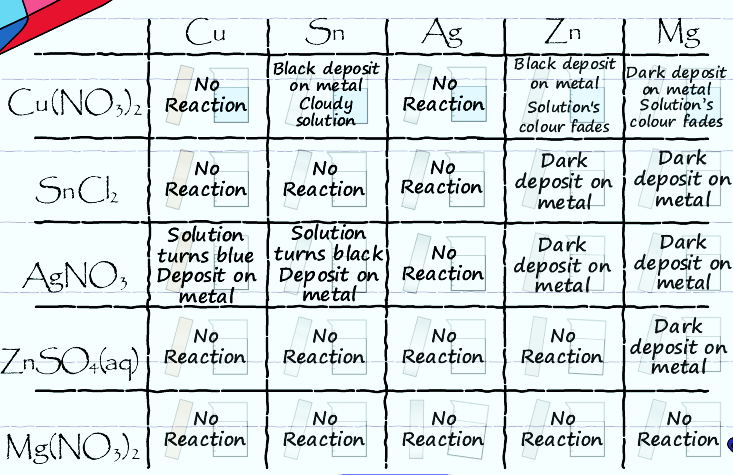# Electrochemistry

-Introduction

Step 1:

-Investigating redox reactions

-Practice with redox reactions

-Reduction tendencies of metal ions

Step 2:

-Electron transfer

-Electrochemical cell

-Practice with cells

-Powering a stopwatch

Step 3:

-Measuring potentials

-Calculating potentials

-Practice with potentials

-Applying potentials

Step 4:

-Non-standard conditions

-Practice with non-standard cells

Feedback Form:

Electrochemistry Tutorial: Galvanic Cells and the Nernst Equation >> Step 1: Practice with redox reactions

 < Previous | Next >

# Electrochemistry: Galvanic Cells and the Nernst Equation

## Step 1: - Practice with redox reactions

After completing the activity on the previous page, you saw that the following table of data was collected from the experiments.Use the data in this table to answer the following questions about how the metal ions reacted.

Based on the table of data, can you arrange the metal ions in the list below from the ion that has the highest tendency to undergo reduction to ion that has the least tendency to undergo reduction?

The highest tendency to undergo reduction

The lowest tendency to undergo reduction

Zn2+
Cu2+
Sn2+
Mg2+
Ag+
Hint
Looking at the table, which metal ion plated out on every other metal? Silver ion plated out as silver on every other metal, so it is the most reactive. Refer back to the table to determine which metal ions are more and less reactive. The order of metal ions from most to least reactive is: Ag+, Cu2+, Sn2+, Zn2+ and Mg2+.

 < Previous | Next >